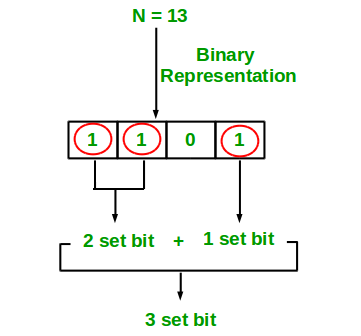# C# Program for Count set bits in an integer

• Last Updated : 02 Jan, 2019

Write an efficient program to count number of 1s in binary representation of an integer.

Examples :

```Input : n = 6
Output : 2
Binary representation of 6 is 110 and has 2 set bits

Input : n = 13
Output : 3
Binary representation of 11 is 1101 and has 3 set bits
```## Recommended: Please solve it on “PRACTICE ” first, before moving on to the solution.

1. Simple Method Loop through all bits in an integer, check if a bit is set and if it is then increment the set bit count. See below program.

## C#

 `// C# program to Count set``// bits in an integer``using` `System;`` ` `class` `GFG {``    ``// Function to get no of set``    ``// bits in binary representation``    ``// of positive integer n``    ``static` `int` `countSetBits(``int` `n)``    ``{``        ``int` `count = 0;``        ``while` `(n > 0) {``            ``count += n & 1;``            ``n >>= 1;``        ``}``        ``return` `count;``    ``}`` ` `    ``// Driver Code``    ``public` `static` `void` `Main()``    ``{``        ``int` `i = 9;``        ``Console.Write(countSetBits(i));``    ``}``}`` ` `// This code is contributed by Sam007`

Output:

```2
```

Recursive Approach :

## C#

 `// C# implementation of recursive``// approach to find the number of``// set bits in binary representation``// of positive integer n``using` `System;`` ` `class` `GFG {`` ` `    ``// recursive function``    ``// to count set bits``    ``public` `static` `int` `countSetBits(``int` `n)``    ``{`` ` `        ``// base case``        ``if` `(n == 0)``            ``return` `0;`` ` `        ``else`` ` `            ``// if last bit set``            ``// add 1 else add 0``            ``return` `(n & 1) + countSetBits(n >> 1);``    ``}`` ` `    ``// Driver code``    ``static` `public` `void` `Main()``    ``{`` ` `        ``// get value``        ``// from user``        ``int` `n = 9;`` ` `        ``// function calling``        ``Console.WriteLine(countSetBits(n));``    ``}``}`` ` `// This code is contributed by aj_36`

Output:

```2
```

Please refer complete article on Count set bits in an integer for more details!

My Personal Notes arrow_drop_up